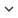ENGArticle
Article 39
Author/s
• M. Fernández-Delgado, J. Ribeiro, E. Cernadas, S. Barro
Source
• IEEE Transactions on Neural Networks, 2011

# Direct Parallel Perceptrons (DPPs): Fast Analytical Calculation of the Parallel PerceptronsWeights with Margin Control for Classification Tasks

Parallel perceptrons (PPs) are very simple and efficient committee machines (a single layer of perceptrons with threshold activation functions and binary outputs, and a majority voting decision scheme), which nevertheless behave as universal approximators. The parallel delta (P-Delta) rule is an effective training algorithm, which, following the ideas of statistical learning theory used by the support vector machine (SVM), raises its generalization ability by maximizing the difference between the perceptron activations for the training patterns and the activation threshold (which corresponds to the separating hyperplane). In this paper, we propose an analytical closedform expression to calculate the PPs’ weights for classification tasks. Our method, called Direct Parallel Perceptrons (DPPs), directly calculates (without iterations) the weights using the training patterns and their desired outputs, without any search or numeric function optimization. The calculated weights globally minimize an error function which simultaneously takes into account the training error and the classification margin. Given its analytical and noniterative nature, DPPs are computationally much more efficient than other related approaches (P-Delta and SVM), and its computational complexity is linear in the input dimensionality. Therefore, DPPs are very appealing, in terms of time complexity and memory consumption, and are very easy to use for high-dimensional classification tasks. On real benchmark datasets with two and multiple classes, DPPs are competitive with SVM and other approaches but they also allow online learning and, as opposed to most of them, have no tunable parameters.
Keywords: Analytical closed-form weight calculation, linear computational complexity, margin maximization, online learning, parallel delta rule, parallel perceptrons, pattern classification